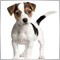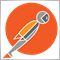Help - Problems with Multi Timeframe Multi Currency Bar Size Alerts152

I have put together  a very simple indicator that creates alerts on bar size of the previous candle in different currencys and different timeframes

THE IDEA:

during the day I wanted to be alerted to large candle sizes across the 28 pairs I monitor

if the last closed candle high to low/ or low to high is

over --  500 / 750 / 1000 or 1500 points  -- a alert is created and also a custom sound file (.wav) played

the indicator seems to work ok, but there are a 2 problems I still have with this

1) I have hard coded in the checks for barsize

dPoint_Diff > 0.01000, dPoint_Diff > 0.01500 etc etc

which causes problems on the JPY pairs as they are 3 decemial points and not 5 decemial points I have hard coded in

2) just doing a simple if check on the TIMEFRAMES  that have been selected to monitor

is not good as I have duplicate code in the if (AlertOnM1) ,if (AlertOnM5) ,if (AlertOnM15) ,if (AlertOnM30) etc etc  blocks

I no its not good to duplicate the code in the blocks over and over, but this does work, but is not ideal

NOTE: on the indicator I would only select 1 or 2 timeframes max to monitor at a time during normal trading hours

example, if I have the 1 min & 5 min  set only to monitor it would generate alerts when events like non farm payrolls occur or other big moves

CODE Attached

Files:
BarSizes.mq5 27 kb152

I think I have fixed the problem with the jpy pairs

I just changed to point check to whole numbers and did a check on 3 or 5 digits on the currency pair

// convert the 3 digit jpy pairs to a whole number
if (getdig(symbols[z]) == 3) {dPoint_Diff *= 1000;}

//convert the 5 digit pairs to a whole number

if (getdig(symbols[z]) == 5) {dPoint_Diff *= 100000;}

if(dPoint_Diff > 1500)
{  // we have a 1500 point bar, now do stuff here }

revised code below152

//+------------------------------------------------------------------+
//|                        Copyright 2018, MetaQuotes Software Corp. |
//|                                             https://www.mql5.com |
//+------------------------------------------------------------------+
#property strict
#property indicator_chart_window
//+------------------------------------------------------------------+
//|                                                                  |
//+------------------------------------------------------------------+
enum tip
{
BarSize,
Other
};
//+------------------------------------------------------------------+
//| 10/12/17 Added in extra alert sounds                             |
//+------------------------------------------------------------------+
input bool   EnableSoundAlerts  = true;
input string s500PointBarSoundFileName  = "500PointBar.wav";
input string s1000PointBarSoundFileName = "1000PointBar.wav";
input string s1500PointBarSoundFileName = "1500PointBar.wav";
//+------------------------------------------------------------------+
//| //END 10/12/17 Added in extra alert sounds                       |
//+------------------------------------------------------------------+

string symbols[];
string      sep=",";
ushort  u_sep=StringGetCharacter(sep,0);
int   symb_cnt=0;
bool newb={false,false,false,false,false,false,false,false};
static datetime lastbar={0,0,0,0,0,0,0,0};
ENUM_TIMEFRAMES tfs=
{
PERIOD_M1,
PERIOD_M5,
PERIOD_M15,
PERIOD_M30,
PERIOD_H1,
PERIOD_H4,
PERIOD_D1,
PERIOD_W1,
PERIOD_MN1,

};

double tbprice=0;
datetime tbtime=0;
//+------------------------------------------------------------------+
//| Custom indicator initialization function                         |
//+------------------------------------------------------------------+
int OnInit()
{symb_cnt=StringSplit(Symbols,u_sep,symbols);

for(int z=0;z<ArraySize(symbols);z++)

lastbar=0;
lastbar=0;
lastbar=0;
lastbar=0;
lastbar=0;
lastbar=0;
lastbar=0;
lastbar=0;
lastbar=0;
//--- indicator buffers mapping
EventSetTimer(1);
//---
return(INIT_SUCCEEDED);
}
//+------------------------------------------------------------------+
//|                                                                  |
//+------------------------------------------------------------------+
void OnDeinit(const int reason)
{
Comment("");
EventKillTimer();
}
//+------------------------------------------------------------------+
//| Custom indicator iteration function                              |
//+------------------------------------------------------------------+
int OnCalculate(const int rates_total,
const int prev_calculated,
const datetime &time[],
const double &open[],
const double &high[],
const double &low[],
const double &close[],
const long &tick_volume[],
const long &volume[],
{

if(MQLInfoInteger(MQL_TESTER))
Process();
//---

//--- return value of prev_calculated for next call
return(rates_total);
}
//+------------------------------------------------------------------+
//| Timer function                                                   |
//+------------------------------------------------------------------+
void OnTimer()
{
if(!MQLInfoInteger(MQL_TESTER))
Process();
//---

}
void Process()
{
for(int s=0;s<9;s++)
{
for(int z=0;z<ArraySize(symbols);z++)
{

{

MqlRates rates[];
ArraySetAsSeries(rates,true);
// Get the open close hi and low of the last 2 bars into rates[] array
CopyRates(symbols[z],PERIOD_M1,0,2,rates);

// rates[i].open
// rates[i].high
// rates[i].low
// rates[i].close
// rates[i].tick_volume

// Point Differance between bars
static double dPoint_Diff;

// Current Barsize is greater than last Barsize - GOING UP
if(rates.high > rates.low)
{

// Differance in points between the high and low of last bar
dPoint_Diff   = rates.high - rates.low;

// convert the 3 digit jpy pairs to a whole number
if (getdig(symbols[z]) == 3) {dPoint_Diff *= 1000;}
//convert the 5 digit pairs to a whole number
if (getdig(symbols[z]) == 5) {dPoint_Diff *= 100000;}

if(dPoint_Diff > 1500)
{

//Alert_(symbols[z]+" p-"+getdig(symbols[z])+" h-"+rates.high+" l-"+rates.low+"<<< 1500 buyxxx alert happend here on: Diff =  "+DoubleToString(dPoint_Diff,getdig(symbols[z])));
Alert_(symbols[z]+" <<< 1500 buy alert happend here on: Diff =  "+DoubleToString(dPoint_Diff,getdig(symbols[z])));

}
else if(dPoint_Diff > 1000)
{

Alert_(symbols[z]+" << 1000 buy alert happend here on: Diff =  "+DoubleToString(dPoint_Diff,getdig(symbols[z])));

}
else if(dPoint_Diff > 750)
{

Alert_(symbols[z]+" < 750 buy alert happend here on: Diff =  "+DoubleToString(dPoint_Diff,getdig(symbols[z])));

}
else if(dPoint_Diff > 500)
{

Alert_(symbols[z]+" b500 buy alert happend here on: Diff =  "+DoubleToString(dPoint_Diff,getdig(symbols[z])));

}
else
{

}

}

// Current Barsize is less than last Barsize - GOING DOWN
else if(rates.low < rates.high)
{

// Differance in points between the high and low of last bar
dPoint_Diff   = rates.low - rates.high;

// convert the 3 digit jpy pairs to a whole number
if (getdig(symbols[z]) == 3) {dPoint_Diff *= 1000;}
//convert the 5 digit pairs to a whole number
if (getdig(symbols[z]) == 5) {dPoint_Diff *= 100000;}

if(dPoint_Diff > 1500)
{

Alert_(symbols[z]+" >>> 1500 DOWN point bar size: Diff =  "+DoubleToString(dPoint_Diff,getdig(symbols[z])));

}
else if(dPoint_Diff > 1000)
{

Alert_(symbols[z]+" >> 1000 DOWN point bar size: Diff =  "+DoubleToString(dPoint_Diff,getdig(symbols[z])));

}
else if(dPoint_Diff > 750)
{

Alert_(symbols[z]+" > 750 DOWN point bar size: Diff =  "+DoubleToString(dPoint_Diff,getdig(symbols[z])));

}
else if(dPoint_Diff > 500)
{

Alert_(symbols[z]+" 500 DOWN point bar size: Diff =  "+DoubleToString(dPoint_Diff,getdig(symbols[z])));

}
else
{

//Alert_(DoubleToString(dPoint_Diff,getdig(_Symbol))+" e-sell alert happend here on: Diff = "+DoubleToString(dPoint_Diff,getdig(_Symbol)));

}

}

}

}
}
}
//+------------------------------------------------------------------+
//|                                                                  |
//+------------------------------------------------------------------+
bool NewBar(int z)
{
datetime curbar=iTime(NULL,tfs[z],0);
if(lastbar[z]!=curbar)
{
lastbar[z]=curbar;
return (true);
}
else return(false);
}
//+------------------------------------------------------------------+
//|                                                                  |
//+------------------------------------------------------------------+
string  StringTf(ENUM_TIMEFRAMES tf)
{
if(tf==PERIOD_M1)return("M1");
if(tf==PERIOD_M5)return("M5");
if(tf==PERIOD_M15)return("M15");
if(tf==PERIOD_M30)return("M30");
if(tf==PERIOD_H1)return("H1");
if(tf==PERIOD_H4)return("H4");
if(tf==PERIOD_D1)return("D1");
if(tf==PERIOD_W1)return("W1");
if(tf==PERIOD_MN1)return("MN1");

return("");
}

//+--
int getdig(string symb)
{
return(int(SymbolInfoInteger(symb,SYMBOL_DIGITS)));
}
//+------------------------------------------------------------------+
//+------------------------------------------------------------------+
datetime expiredate=0;
string txtname="DTS"+".txt";

//+------------------------------------------------------------------+
{
SendMail(MQLInfoString(MQL_PROGRAM_NAME),text);
}
//+------------------------------------------------------------------+
datetime iTime(string symb,ENUM_TIMEFRAMES timeframe,int nShift)
{
datetime timeseries;
if(CopyTime(symb,timeframe,nShift,1,timeseries)==1) return(timeseries);
else return(-1.0);
}
//+------------------------------------------------------------------+
//|                                                                  |
//+------------------------------------------------------------------+
ENUM_TIMEFRAMES TFMigrate(int tf)
{
switch(tf)
{
case 0: return(PERIOD_CURRENT);
case 1: return(PERIOD_M1);
case 5: return(PERIOD_M5);
case 15: return(PERIOD_M15);
case 30: return(PERIOD_M30);
case 60: return(PERIOD_H1);
case 240: return(PERIOD_H4);
case 1440: return(PERIOD_D1);
case 10080: return(PERIOD_W1);
case 43200: return(PERIOD_MN1);

case 2: return(PERIOD_M2);
case 3: return(PERIOD_M3);
case 4: return(PERIOD_M4);
case 6: return(PERIOD_M6);
case 10: return(PERIOD_M10);
case 12: return(PERIOD_M12);
case 16385: return(PERIOD_H1);
case 16386: return(PERIOD_H2);
case 16387: return(PERIOD_H3);
case 16388: return(PERIOD_H4);
case 16390: return(PERIOD_H6);
case 16392: return(PERIOD_H8);
case 16396: return(PERIOD_H12);
case 16408: return(PERIOD_D1);
case 32769: return(PERIOD_W1);
case 49153: return(PERIOD_MN1);
default: return(PERIOD_CURRENT);
}
}
//+------------------------------------------------------------------+152

I have duplicate code blocks for

but I have removed the from the above code as the character limit was excceded

putting duplicate code blocks in for the checks if 1,5,15,30 minute alerts are enabled does not seem like a good idea

does anyone have a idea which would be the best way to check for which alerts timeframes are enabled, and run threw a single block of code??2391

xop32:

I have duplicate code blocks for

but I have removed the from the above code as the character limit was excceded

putting duplicate code blocks in for the checks if 1,5,15,30 minute alerts are enabled does not seem like a good idea

does anyone have a idea which would be the best way to check for which alerts timeframes are enabled, and run threw a single block of code??

Couple of tips...

This will never resolve to true

rates.low < rates.high

You can greatly simplify your code by using objects. You can create a unique object for each alert.

//+------------------------------------------------------------------+
//|                                      Copyright 2018, nicholishen |
//|                                          http://forexfactory.com |
//+------------------------------------------------------------------+
#property version   "1.00"
#property strict
#property indicator_chart_window

input string InpCurrencies = "AUD,CAD,CHF,JPY,NZD,USD,EUR,GBP";
input string InpTimeframes = "M1,M5,M15,H1";
input string InpPointValues= "50,100,500,1000,1500";
//+------------------------------------------------------------------+
//| Custom indicator initialization function                         |
//+------------------------------------------------------------------+
#include <Tools\DateTime.mqh>
#include <Arrays\ArrayObj.mqh>
#include <Arrays\ArrayInt.mqh>
#include <Arrays\ArrayString.mqh>

class BarAlert : public CObject
{
protected:
string            m_symbol;
ENUM_TIMEFRAMES   m_timeframe;
MqlRates          m_rates[];
double            m_point;
string            m_sound;
public:
BarAlert(const string symbol,const ENUM_TIMEFRAMES timeframe, const uint alert_points, string sound = NULL)
{
SymbolSelect(symbol,true);
m_symbol       = symbol;
m_timeframe    = timeframe;
m_sound        = sound;
m_point        = SymbolInfoDouble(m_symbol,SYMBOL_POINT);
ArraySetAsSeries(m_rates,true);
}
void Refresh()
{
if(CopyRates(m_symbol,m_timeframe,0,1,m_rates) < 1)
{
printf("CopyRates error %d",_LastError);
return;
}
return;
double delta = m_rates.high - m_rates.low;
if(delta >= (double)m_alert_points * m_point)
{
if(m_sound != NULL)
PlaySound(m_sound);
string alert_text = StringFormat("%s: %d point bar size on %s",m_symbol,int(delta/m_point),EnumToString(m_timeframe));
}
}
{
return ArraySize(m_rates)<1? false : m_rates.time == m_last_alert;
}
protected:
{
SendMail(MQLInfoString(MQL_PROGRAM_NAME),text);
}
};

{
protected:
public:
bool OnInit(string currencies, string timeframes,string point_vals)
{
string c[],t[],p[];
ENUM_TIMEFRAMES all_timeframes[] = {PERIOD_M1,PERIOD_M5,PERIOD_M15,PERIOD_M30,PERIOD_H1,PERIOD_H4,PERIOD_D1};
int total_currencies = StringSplit(currencies,',',c);
int total_timeframes = StringSplit(timeframes,',',t);
int total_point_vals = StringSplit(point_vals,',',p);
CArrayInt timeframes_to_use;
for(int i=0;i<total_timeframes;i++)
for(int j=0;j<ArraySize(all_timeframes);j++)
if(StringFind(EnumToString(all_timeframes[j]),t[i]) >= 0)
break;
CArrayString symbols_to_use;
for(int i=0;i<total_currencies;i++)
for(int j=0;j<total_currencies;j++)
{
if(j==i)
continue;
string test_pair = c[i]+c[j];
for(int k=0;k<SymbolsTotal(false);k++)
if(StringFind(SymbolName(k,false),test_pair) >=0)
break;
}
for(int i=0;i<symbols_to_use.Total();i++)
for(int j=0;j<timeframes_to_use.Total();j++)
for(int k=0;k<total_point_vals;k++)
{
}
return true;
}
void Refresh()
{
}
};

int OnInit()
{
//--- indicator buffers mapping
if(!g_manager.OnInit(InpCurrencies,InpTimeframes,InpPointValues))
return INIT_FAILED;
EventSetTimer(1);
//---
return(INIT_SUCCEEDED);
}
//+------------------------------------------------------------------+
//| Custom indicator iteration function                              |
//+------------------------------------------------------------------+
int OnCalculate(const int rates_total,
const int prev_calculated,
const datetime &time[],
const double &open[],
const double &high[],
const double &low[],
const double &close[],
const long &tick_volume[],
const long &volume[],
{
return(rates_total);
}
//+------------------------------------------------------------------+
//| Timer function                                                   |
//+------------------------------------------------------------------+
void OnTimer()
{
g_manager.Refresh();
}
//+------------------------------------------------------------------+152

thanks for the help nicholishen

I will study your code and test it out when the markets open again on tuesday

your code looks a lot cleaner than my attempt at it